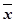# Student Resources

## Glossary

Asymptote: A line that a graph approaches, but does not reach, as x- or y-values increase in a positive or negative direction.

Density Curve: A mathematical model of a distribution. The total area under the curve, by definition, is equal to 1, or 100%. The area under the curve for a range of values is the proportion of all observations for that range.

Distribution: The way in which the data is spread.

Empirical Rule: The empirical rule, also known as the 68-95-99.7 rule, describes the spread of data in a normal distribution given the mean and standard deviation. 68% of the data will fall within one standard deviation of the mean, 95% of the data will fall within two standard deviations of the mean, almost all (99.7%) of the data will fall within three standard deviations of the mean.

Frequency: How often a data point appears in the data set.

Mean: A measure of central tendency for a one-variable data set. It is found by dividing the sum of all values by the number of values.

•(x-bar)   represents the mean of a sample.
• μ (Pronounced "mu" ) represents the mean of a population.

Mean Absolute Deviation: A measure of spread for a one-variable data set that uses absolute value to eliminate the effect of the different signs of the individual deviations.

Normal Curve: The graph of a normal distribution.

Normal Distribution: A symmetric bell-shaped distribution.

Percentile: The percentage of values in a data set that are below a given value.

Population: The whole group from which a sample is taken.

Sample: A selection taken from a larger group, or population, to examine in order to find out characteristics about the population.

Skewed Data: Data that are spread out more on one side of the center than on the other side.

Standard Deviation: A measure of spread for a one-variable data set that uses squaring to eliminate the effect of the different signs of the individual deviations.

• "S"  represents the standard deviation of a sample.
• "σ" (lower case Greek letter "sigma") represents the standard deviation of a population.

Symmetric Data: Data that are balanced about the center.